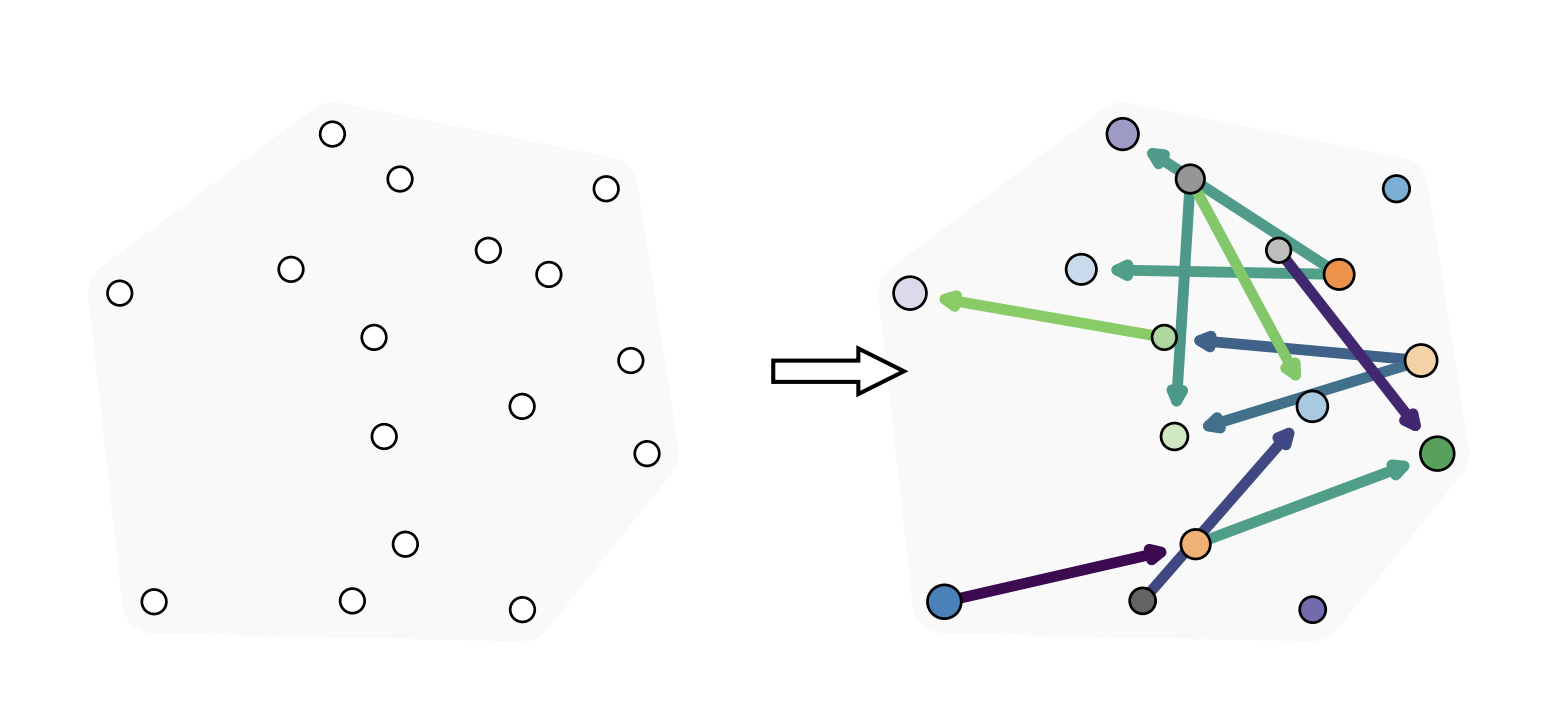# Set2Graph: Learning Graphs From SetsMany problems in machine learning can be cast as learning functions from sets to graphs, or more generally to hypergraphs; in short, Set2Graph functions. Examples include clustering, learning vertex and edge features on graphs, and learning features on triplets in a collection. A natural approach for building Set2Graph models is to characterize all linear equivariant set-to-hypergraph layers and stack them with non-linear activations. This poses two challenges: (i) the expressive power of these networks is not well understood; and (ii) these models would suffer from high, often intractable computational and memory complexity, as their dimension grows exponentially. This paper advocates a family of neural network models for learning Set2Graph functions that is both practical and of maximal expressive power (universal), that is, can approximate arbitrary continuous Set2Graph functions over compact sets. Testing these models on different machine learning tasks, mainly an application to particle physics, we find them favorable to existing baselines.

Authors:
Hadar Serviansky (Weizmann Institute of Science)
Nimrod Segol (Weizmann Institute of Science)
Jonathan Shlomi (Weizmann Institute of Science)
Kyle Cranmer (New York University)
Eilam Gross (Weizmann Institute of Science)
Yaron Lipman (Weizmann Institute of Science)
Publication Date:
Wednesday, December 9, 2020